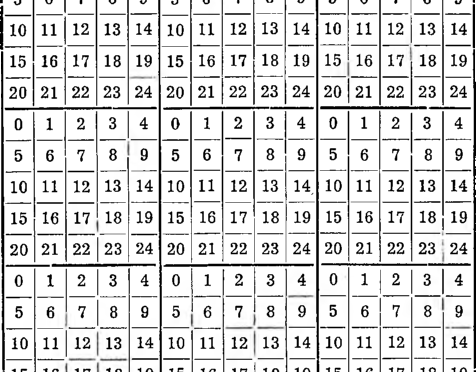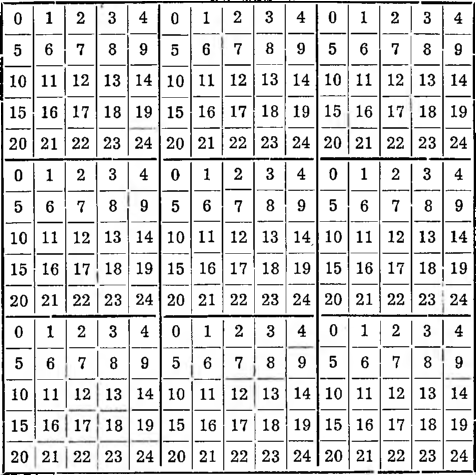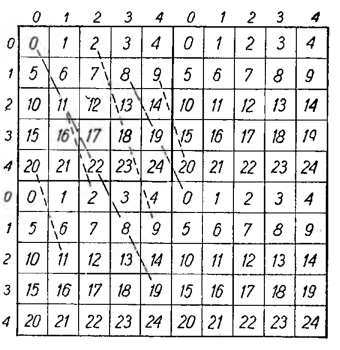# Arithmetic lines part 1

Arithmetic lines

Before we proceed to discuss the hypermagic squares, we need to give some information about arithmetic lines.

Let's look carefully at the next table; it consists of 9 twenty-five-field squares, with consecutively inscribed numbers from 0 do 24.

Instead 9 squares could be taken 16, 25, . . . squares, one could also take non-25 squares, but with a different number of fields, because it is actually not about- their quantity and quality, but to produce some background from a sequence of numbers arranged in squares, on which background we can draw the so-called arithmetic lines and explain their meaning.Without using a ruler and a pencil, you can see one of the arithmetic lines at once, namely, a diagonal extending from, for example, the field occupied by 0 across the field 6, further 12, 18, 24,. . .

Here we will immediately note a peculiarity of this lineage.

A series of numbers, through which the arithmetic line runs

0, 6, 12, 18, 24, …

can be presented as such:

0, 1 + 5, 2 + 2 • 5, 3 + 3 • 5, 4 + 4 • 5, …

So if we denote the output field of a line with 0, then the contents of the first field it cuts across will be 1 + multiple 5, the second: 2 + multiple 5, the third: 3 + multiple 5, fourth: 4 + multiple 5 and so on.

If instead of drawing the diagonal of a square in your imagination, we will take a ruler and a pencil and connect, for example, the center of the field occupied by 0 with the center of the field in the same square by 16, then we will see, that simple, which we will delete and extend further, it will pass through the very center of many

other fields and a sequence of numbers will be created again:

0, 16, 7, 23, 14, …,

which can also be written like this:

0, 1 + 3 • 5, 2 + 1 • 5, 3 + 4 • 5, 4+2 • 5, …

So we get the same again, as before, namely: a sequence of numbers entered in the fields, through the centers of which this arithmetic line runs, it consists of the ordinal numbers of the fields: 0, 1, 2, 3, 4, … plus some multiples 5.

So such straight lines passing through the centers of a series of fields are called arithmetic lines. We were constantly dealing with the magic figures discussed previously (without using that name) with three types of arithmetic lines, which in magic squares were also magic lines, namely with the horizontal lines of the rows of fields, vertical lines of columns and diagonals.

So now we have only extended our interest to other diagonal lines, which among the squares can be carried out, and called all these lines arithmetic lines.Let's take a closer look at the picture below, on which graphically 0 several were carried out 1 arithmetic lines. Distance between adjacent centers- 3 the fields on the line 4 arithmetic is called the step of the arithmetic line.

If along the edges straight- 1 number the rows at the corner 2 fields and columns (as he sees it- 3 we are in the picture), it's each 4 the field can be numbered as a cross between the corresponding column and row, always putting the column number first, on the second - row number. For example, in the field (2,3) there is a number 17, a na polu (3,2) - number 13.